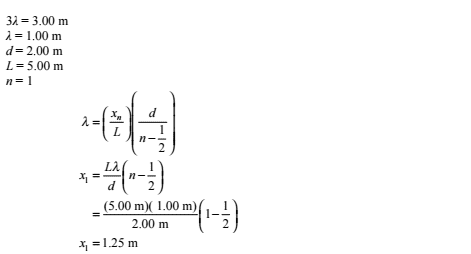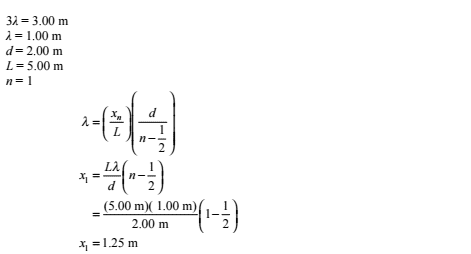# Wave interference question

## Homework Statement

In a large water tank experiment, water waves are generated with straight, parallel wave fronts, 3.00 m apart. The wave fronts pass through two openings 5.00 m apart in a long board. The end of the tank is 3.00 m beyond the board. Where would you stand, relative to the perpendicular bisector of the line between the openings, if you want to receive little or no wave action?

## Homework Equations

Xn/L = (n-0.5)wavelength/d

n is the number of the nodal line, xn is the distance from the bisector to the point on the nodal line, d is the distance between both sources and L is the distance from the midpoint of both sources to the point on the nodal line.

## The Attempt at a Solution

For little to no wave action I know the person has to be standing on a nodal line, and the distance from the right bisector to that point would be Xn.

What I've gathered from the question is that the wave length is 3m, d=5m, and L = 3m, and I think n is 1.
However, when I use these numbers i get an answer of 0.9m, when the answer in the back of the book is 1.25m.

This is the answer in the solution manual and I have no clue how they got the wavelength to be 1m, the d to be 2m and the L to be 5m. Help is appreciated.TSny
Homework Helper
Gold Member

## Homework Equations

Xn/L = (n-0.5)wavelength/dIt does appear that the solution is for a different set of data.

The equation Xn/L = (n-0.5)wavelength/d, which is equivalent to the equation used in the solution, is accurate only for "small angles". It would be better to use formulas that don't assume small angles. For example, using the data in the solution, the more accurate answer would be 1.29 m rather than 1.25 m.

It does appear that the solution is for a different set of data.

The equation Xn/L = (n-0.5)wavelength/d, which is equivalent to the equation used in the solution, is accurate only for "small angles". It would be better to use formulas that don't assume small angles. For example, using the data in the solution, the more accurate answer would be 1.29 m rather than 1.25 m.

Were the values I concluded from the problem correct?, and what formula was used to get the answer of 1.29m?

TSny
Homework Helper
Gold Member
If the screen is "far" from the slits (L >> d) then there is a formula for the angle θ representing the direction to the first minimum (destructive interference). This formula is based on the "path difference" from the two slits being approximated as d⋅sin θ. Using the condition on the path difference for a minimum, you can calculate θ. Then, using geometry, you can find the distance from the perpendicular bisector to the location of the minimum at the end of the tank.

This is how I got my result of 1.29 m. However, I now realize that the assumption L >> d does not hold in your problem. So, d⋅sin θ is not accurate for the path difference.

Using geometry you can find an accurate expression for the path difference, but it is more complicated. If I redo the calculation without any approximations, I get 1.31 m for the data given in the solution.

Since the solution uses the approximate formula, I would guess that you are OK with working the problem as you did. But, keep in mind that your answer is only an approximation. Without approximation, I get 1.21 m instead of 0.90 m. So, you can see that your approximated answer is not very accurate.